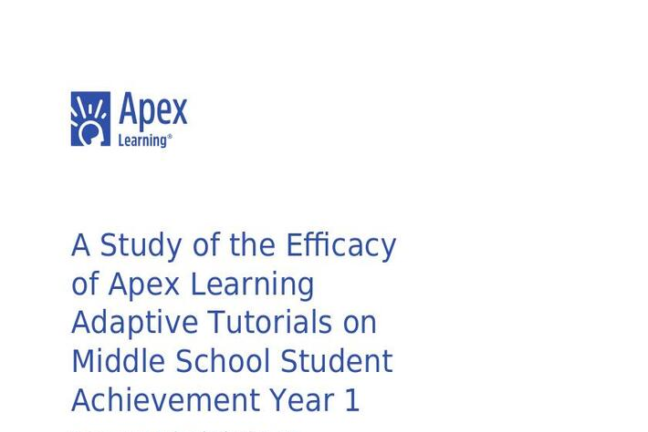Efficacy Research

# A Study of the Efficacy of Apex Learning Adaptive Tutorials on Middle School Student Achievement Year 1Overall, students using Adaptive Tutorials were 13% more likely (.88 / .78) to meet or exceed the state standard on the SBAC assessments than students participating in classroom instruction alone (OR=2.06, p<.03, ES=.44). By subject, students using Tutorials were 20% more likely (.88 / .73) to meet or exceed the state standard for English language arts (OR=2.73, p<.03, ES=.61) and 6% more likely (.87 / .82) for mathematics (OR=1.48, p<.10, ES=.24) than similar students participating in traditional classroom instruction alone. xtagstartz/p>

The percent of students meeting or exceeding the standard on assessments overall was 10 percentage points greater (.88 - .78) for those using Tutorials than similar students not using Tutorials (z=3.29, p<.00). By subject, the percent of students meeting or exceeding the standard on the English language arts assessments was 15 percentage points greater (.88 - .73) for students using Tutorials than students not using Tutorials (z=2.95, p<.00). The difference between the percent of students meeting or exceeding the standard for mathematics trended higher (.87 -. 82) for students using Tutorials than for students not using Tutorials and approached statistical significance (z=1.30, p<.10).

Overall, the average student using Adaptive Tutorials in addition to classroom instruction (M=.61, SD=.65) performed significantly better on the SBAC summative assessment than the average student participating in traditional classroom instruction alone (M=.49, SD=.94) (t(601)=2.99, p<.00, ES=.12).

Students using Tutorials achieved an additional 4 percentile points on the SBAC summative assessments combined and an additional 11 percentile points on the English language arts assessments compared to similar students (t(601)=4.15, p<.00, ES=.30). Students using Tutorials for math achieved similar average scores on the mathematics assessments as students participating in traditional classroom instruction alone.